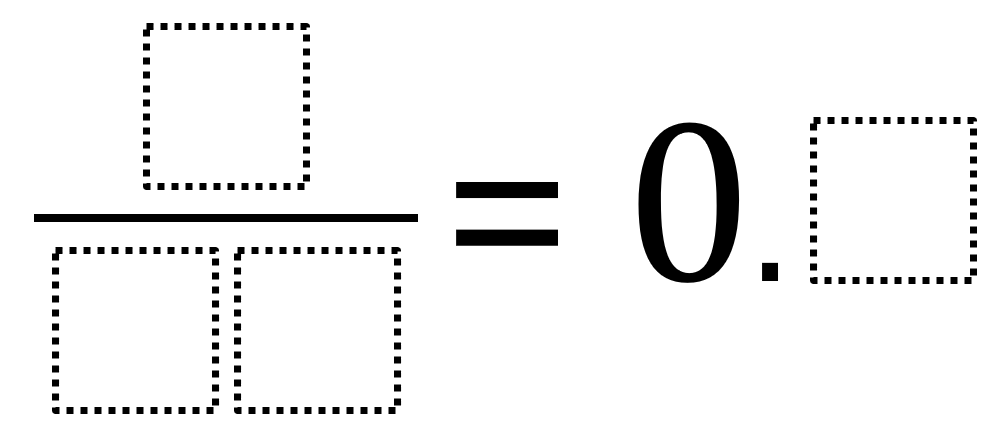Home > Grade 7 > Converting a Fraction to a Decimal

# Converting a Fraction to a Decimal

Directions: Using the digits 1 to 9, at most one time each, place a digit in each box to make a true statement.### Hint

How does choosing the digits for the denominator affect the decimal value?
How might choosing the digit for the decimal make finding the digits for the fraction easier?

There are many possible answers including:
9/18 = 0.5
7/14 = 0.5
6/12 = 0.5
And 7/35 = 0.2

Source: Owen Kaplinsky

## Sides of a Triangle

Directions: The perimeter of a triangle is 20 units. Using whole numbers, how many sets …

1.•2.nice problem! the answers I get are:

0.1 – not possible
0.2 – 3/15 or 7/35
0.3 – not possible
0.4 – 6/15
0.5 – 6/12, 7/14, 8/16, 9/18
0.6 – 9/15
0.7 – not possible
0.8 – not possible
0.9 – not possible

•Some of my students also found 9/45 = 0.2 🙂

3.Great problem!

4.5.1/10= 0.1

6.I think it’s 1/10 = 0.1

7.” how does choosing the digits for the denominator affect the decimal value.” The denominator has to be able to be divided by ten. The fraction in it lowest form has to be able to be converted into its lowest tenths. ” how might choosing the digit for the decimal make finding the digit for the fraction easier”.The decimal amount can just get put over a tenth.

8.